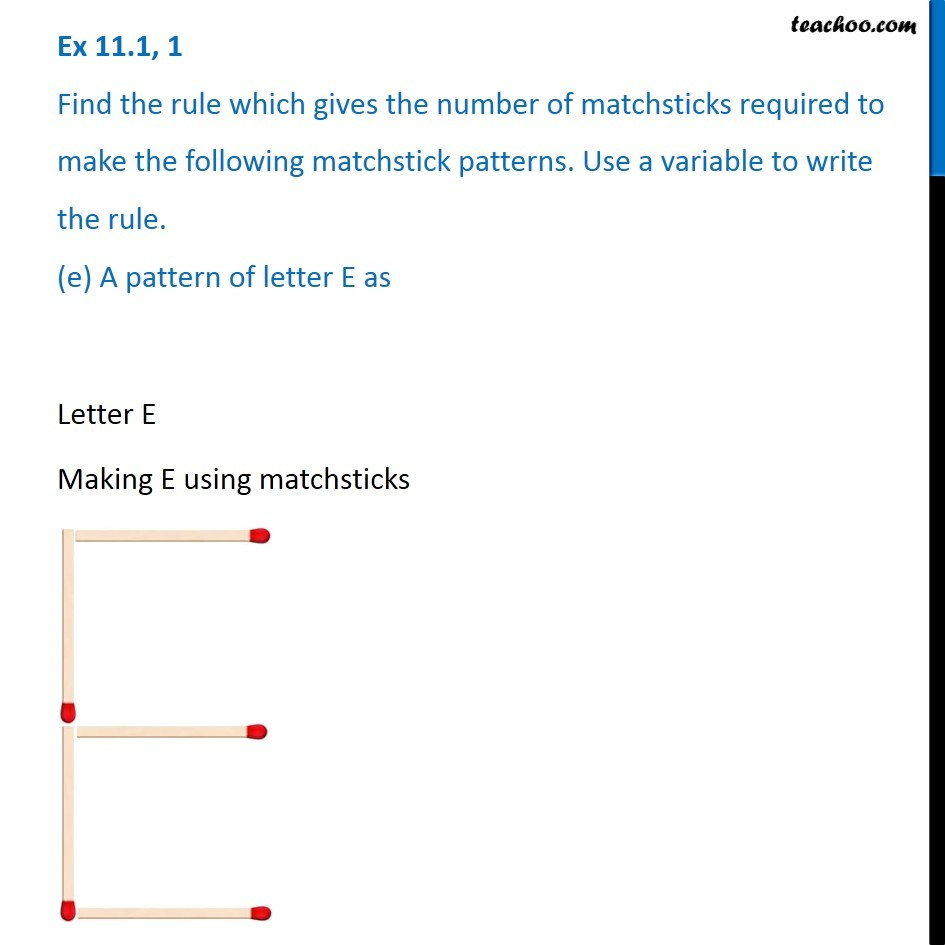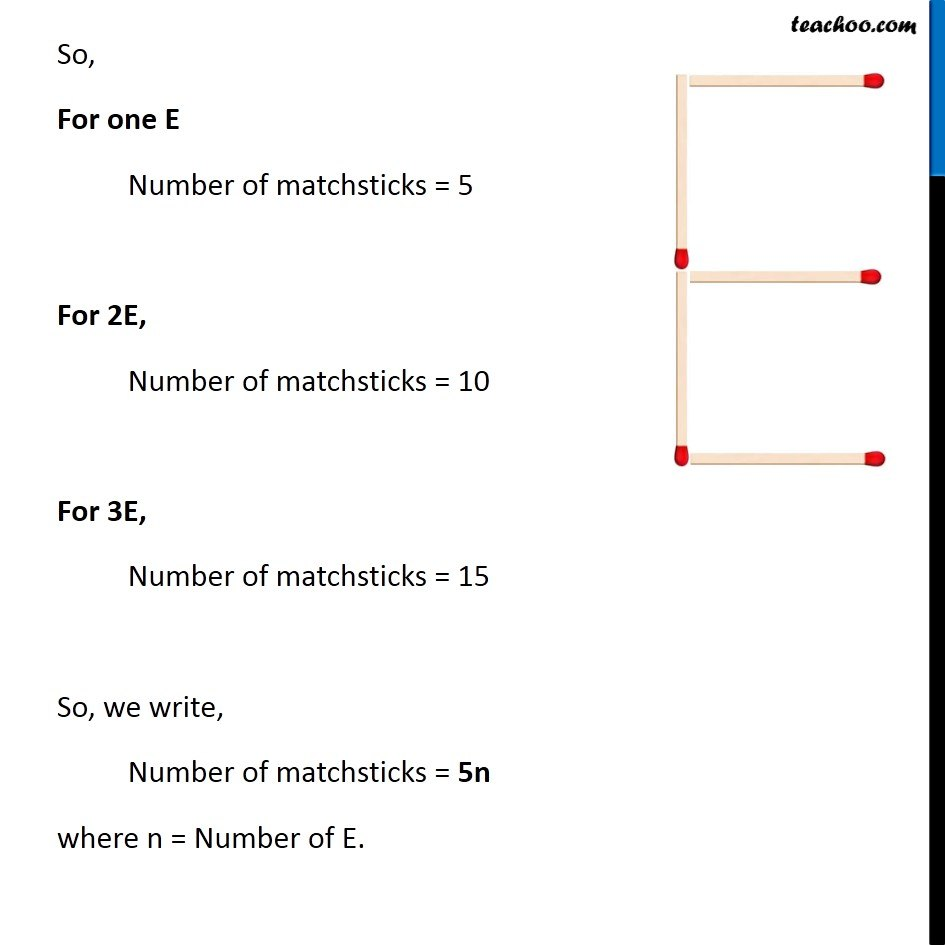Ex 11.1

Chapter 11 Class 6 Algebra
Serial order wiseLearn in your speed, with individual attention - Teachoo Maths 1-on-1 Class

### Transcript

Ex 11.1, 1 Find the rule which gives the number of matchsticks required to make the following matchstick patterns. Use a variable to write the rule. (e) A pattern of letter E asLetter E Making E using matchsticks So, For one E Number of matchsticks = 5 For 2E, Number of matchsticks = 10 For 3E, Number of matchsticks = 15 So, we write, Number of matchsticks = 5n where n = Number of E.